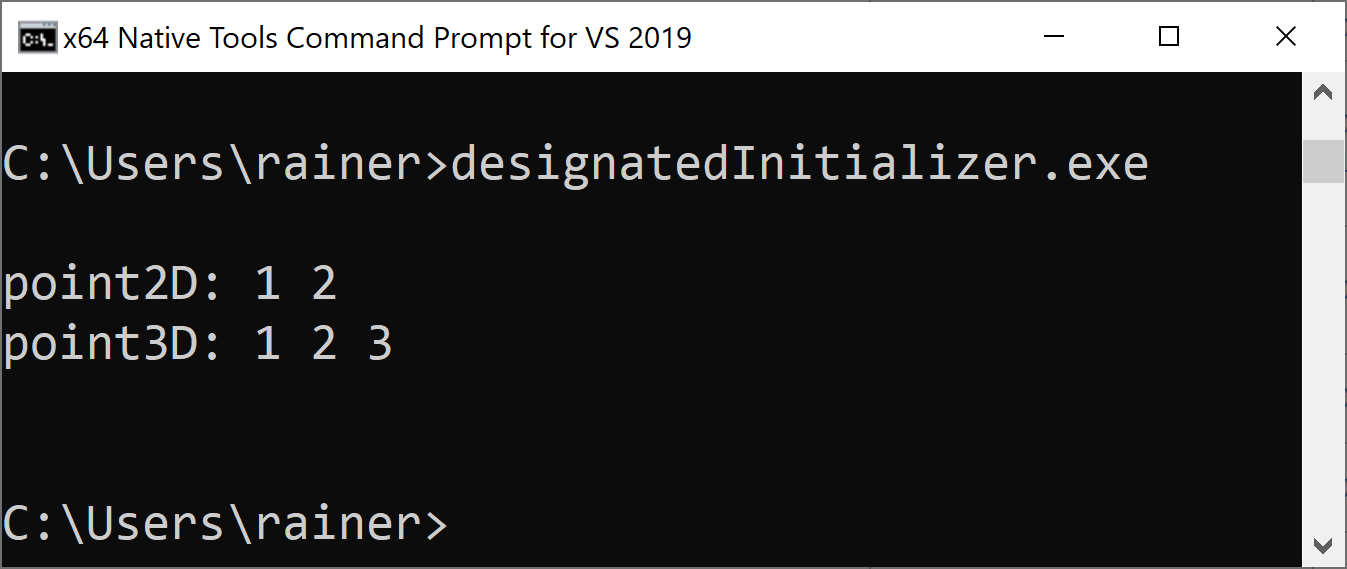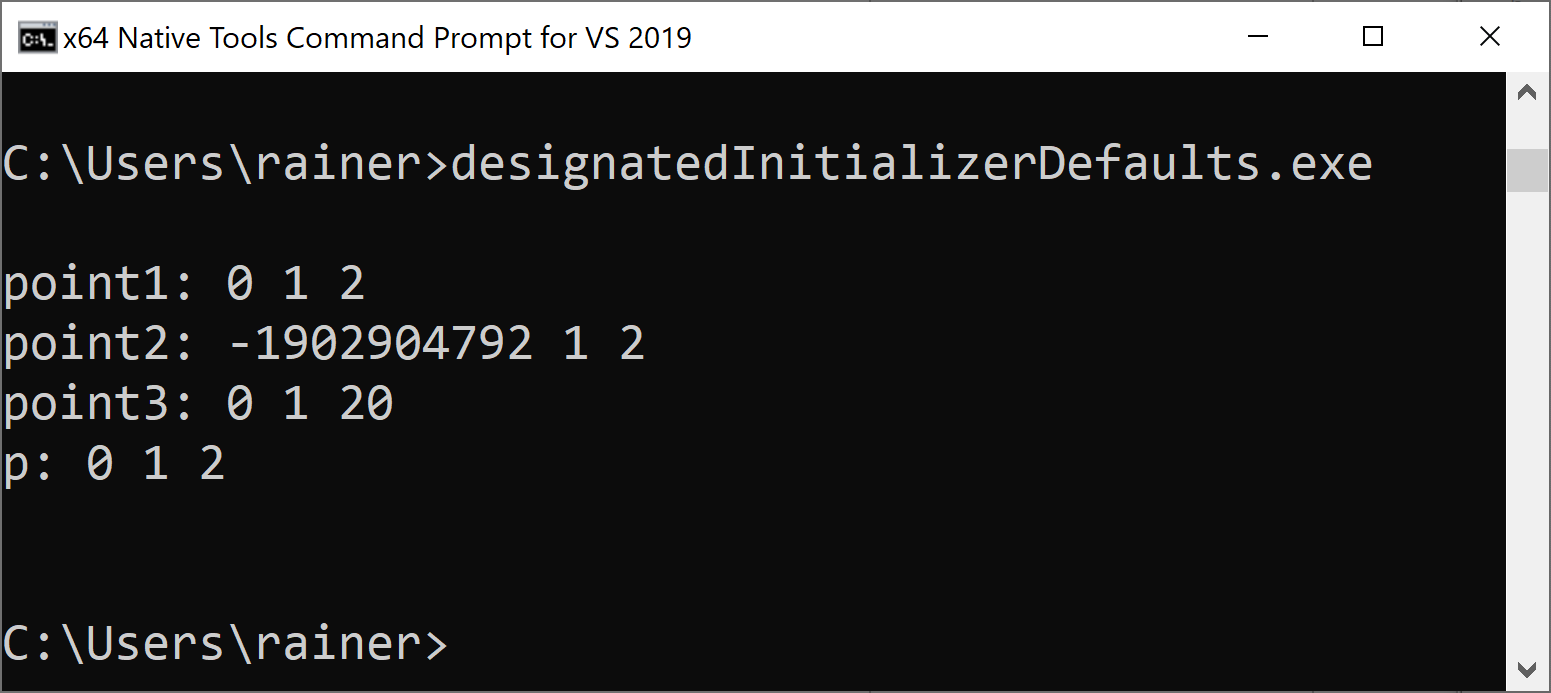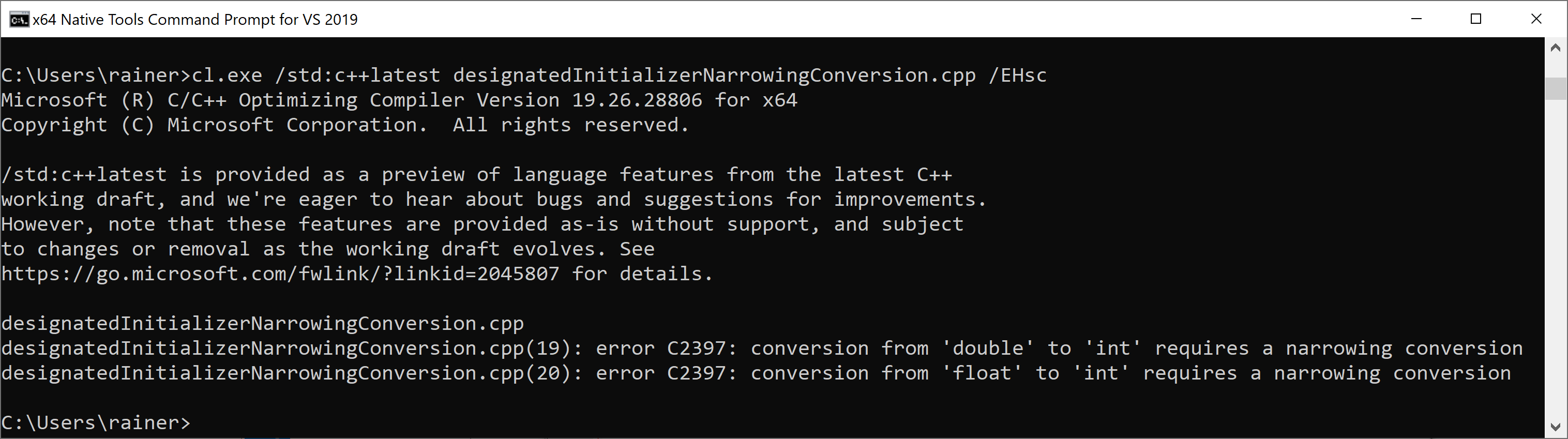# Designated Initializers

Designated initialization is an extension of aggregate initialization and empowers you to directly initialize the members of a class type using their names.Designated initialization is a special case of aggregate initialization. Writing about designated initialization means, therefore, writing about aggregate initialization.

## Aggregate Initialization

First: what is an aggregate? Aggregates are arrays and class types. A class type is a class, a struct, or a union.

With C++20, the following condition must hold class types:

• no private or protected non-static data members
• no user-declared or inherited constructors
• no virtual, private, or protected base classes
• no virtual member functions

The next program exemplifies aggregate initialization.

##Modernes C++ Mentoring

Be part of my mentoring programs:

• "Fundamentals for C++ Professionals" (open)
• "Design Patterns and Architectural Patterns with C++" (open)
• "C++20: Get the Details" (reopens December 2023)
• Do you want to stay informed about my mentoring programs: Subscribe via E-Mail.

```// aggregateInitialization.cpp

#include <iostream>

struct Point2D{
int x;
int y;
};

class Point3D{
public:
int x;
int y;
int z;
};

int main(){

std::cout << std::endl;

Point2D point2D{1, 2};        // (1)
Point3D point3D{1, 2, 3};     // (2)

std::cout << "point2D: " << point2D.x << " " << point2D.y << std::endl;
std::cout << "point3D: " << point3D.x << " " << point3D.y << " " << point3D.z << std::endl;

std::cout << std::endl;

}
```

(1) and (2) directly initialize the aggregates using curly braces. The sequence of the initializers in the curly braces has to match the declaration order of the members.Based on aggregate initialization in C++11, we get designed initializers in C++20. So far, only the Microsoft compiler support designated initializers completely.

## Designated Initializers

Designated initializers enable it to initialize members of a class type using their name directly. For a union, only one initializer can be provided. As for aggregate initialization, the sequence of initializers in the curly braces has to match the declaration order of the members.

```// designatedInitializer.cpp

#include <iostream>

struct Point2D{
int x;
int y;
};

class Point3D{
public:
int x;
int y;
int z;
};

int main(){

std::cout << std::endl;

Point2D point2D{.x = 1, .y = 2};          // (1)
Point3D point3D{.x = 1, .y = 2, .z = 3};  // (2)

std::cout << "point2D: " << point2D.x << " " << point2D.y << std::endl;
std::cout << "point3D: " << point3D.x << " " << point3D.y << " " << point3D.z << std::endl;

std::cout << std::endl;

}
```

(1) and (2) use designated initializers to initialize the aggregates. The initializers, such as .x or .y are often called designators.The members of the aggregate can already have a default value. This default value is used when the initializer is missing. This does not hold for a union.

```// designatedInitializersDefaults.cpp

#include <iostream>

class Point3D{
public:
int x;
int y = 1;
int z = 2;
};

void needPoint(Point3D p) {
std::cout << "p: " << p.x << " " << p.y << " " << p.z << std::endl;
}

int main(){

std::cout << std::endl;

Point3D point1{.x = 0, .y = 1, .z = 2};     // (1)
std::cout << "point1: " << point1.x << " " << point1.y << " " << point1.z << std::endl;

Point3D point2;                             // (2)
std::cout << "point2: " << point2.x << " " << point2.y << " " << point2.z << std::endl;

Point3D point3{.x = 0, .z = 20};            // (3)
std::cout << "point3: " << point3.x << " " << point3.y << " " << point3.z << std::endl;

// Point3D point4{.z = 20, .y = 1}; ERROR   // (4)

needPoint({.x = 0});                        // (5)

std::cout << std::endl;

}
```

(1) initializes all members,  but (2) does not provide a value for member x. Consequently, x is not initialized. It is fine if you only initialize the members who don’t have a default value such as in (3) or (5). The expression (4) would not compile because z and y are in the wrong order.Designated initializers detect narrowing conversion. Narrowing conversion is a conversion of a value, including the loss of its precision.

```// designatedInitializerNarrowingConversion.cpp

#include <iostream>

struct Point2D{
int x;
int y;
};

class Point3D{
public:
int x;
int y;
int z;
};

int main(){

std::cout << std::endl;

Point2D point2D{.x = 1, .y = 2.5};            // (1)
Point3D point3D{.x = 1, .y = 2, .z = 3.5f};   // (2)

std::cout << "point2D: " << point2D.x << " " << point2D.y << std::endl;
std::cout << "point3D: " << point3D.x << " " << point3D.y << " " << point3D.z << std::endl;

std::cout << std::endl;

}
```

(1) and (2) produce a compile-time error because the initialization .y = 2.5 and .z = 3.5f would cause a narrowing conversion to in.Interestingly, designated initializers in C behave differently to designated initializers in C++.

### Differences between C and C++

C supports use cases that are not supported in C++. C allows

• to initialize the members of the aggregate out-of-order
• to initialize the members of a nested aggregate
• to mix designated initializers and regular initializers
• designated initialization of arrays

Proposal P0329R4 provides self-explanatory examples for these use cases:

```struct A { int x, y; };
struct B { struct A a; };
struct A a = {.y = 1, .x = 2}; // valid C, invalid C++ (out of order)
int arr = { = 5};        // valid C, invalid C++ (array)
struct B b = {.a.x = 0};       // valid C, invalid C++ (nested)
struct A a = {.x = 1, 2};      // valid C, invalid C++ (mixed)
```

The rationale for this difference between C and C++ is also part of the proposal: “In C++, members are destroyed in reverse construction order and the elements of an initializer list are evaluated in lexical order, so field initializers must be specified in the order. Array designators conflict with ​lambda-expression​ syntax. Nested designators are seldom used.” The paper argues that only out-of-order initialization of aggregate is commonly used.

## What’s next?

Wow! With C++98, we got const, with C++11 constexpr, and with C++20 consteval and constinit.  In my next post, I will write about the new C++20 specifiers consteval and constinit and their differences from const and constexpr

Thanks a lot to my Patreon Supporters: Matt Braun, Roman Postanciuc, Tobias Zindl, G Prvulovic, Reinhold Dröge, Abernitzke, Frank Grimm, Sakib, Broeserl, António Pina, Sergey Agafyin, Андрей Бурмистров, Jake, GS, Lawton Shoemake, Jozo Leko, John Breland, Venkat Nandam, Jose Francisco, Douglas Tinkham, Kuchlong Kuchlong, Robert Blanch, Truels Wissneth, Kris Kafka, Mario Luoni, Friedrich Huber, lennonli, Pramod Tikare Muralidhara, Peter Ware, Daniel Hufschläger, Alessandro Pezzato, Bob Perry, Satish Vangipuram, Andi Ireland, Richard Ohnemus, Michael Dunsky, Leo Goodstadt, John Wiederhirn, Yacob Cohen-Arazi, Florian Tischler, Robin Furness, Michael Young, Holger Detering, Bernd Mühlhaus, Matthieu Bolt, Stephen Kelley, Kyle Dean, Tusar Palauri, Dmitry Farberov, Juan Dent, George Liao, Daniel Ceperley, Jon T Hess, Stephen Totten, Wolfgang Fütterer, Matthias Grün, Phillip Diekmann, Ben Atakora, Ann Shatoff, Rob North, and Bhavith C Achar.

Thanks, in particular, to Jon Hess, Lakshman, Christian Wittenhorst, Sherhy Pyton, Dendi Suhubdy, Sudhakar Belagurusamy, Richard Sargeant, Rusty Fleming, John Nebel, Mipko, Alicja Kaminska, Slavko Radman, and David Poole.

 My special thanks to EmbarcaderoMy special thanks to PVS-StudioMy special thanks to Tipi.buildMy special thanks to Take Up Code## Seminars

I’m happy to give online seminars or face-to-face seminars worldwide. Please call me if you have any questions.

### Standard Seminars (English/German)

Here is a compilation of my standard seminars. These seminars are only meant to give you a first orientation.

• C++ – The Core Language
• C++ – The Standard Library
• C++ – Compact
• C++11 and C++14
• Concurrency with Modern C++
• Design Pattern and Architectural Pattern with C++
• Embedded Programming with Modern C++
• Generic Programming (Templates) with C++

#### New

• Clean Code with Modern C++
• C++20

### Contact Me

Modernes C++ Mentoring,Tags:
0 replies